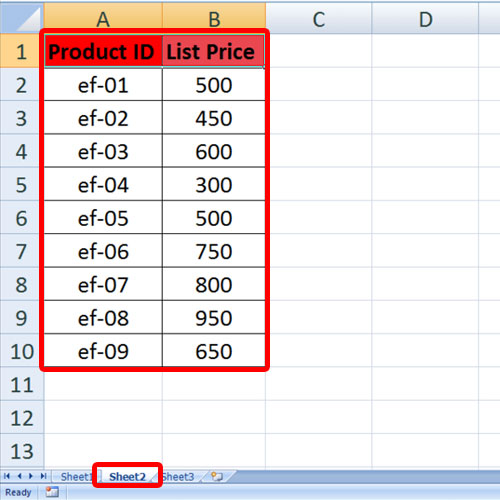## How to Copy or Paste Data in Excel via VBA

In this tutorial we will guide you on how to copy and paste data in Excel using VBA. Copying data and pasting it into another cell or worksheet is a simple task in Microsoft Excel. However it can trouble you a lot if you have a large amount of data and multiple spreadsheets to work with. Visual Basic for applications i.e. VBA helps you to perform these kinds of tasks which you need to carry out repeatedly. So here we are creating a VBA copy and VBA paste scripts using Excel macros for copying and pasting of data from one Sheet to another.

You will find a Script example in the end of this lesson.

Step 1 – Open up the data

To create VBA copy and VBA paste script, we have opened up a worksheet that contains 2 sheets. The first sheet has all the data entered and the second sheet is basically empty. So let’s copy the data from sheet 1 to sheet 2 using VBA.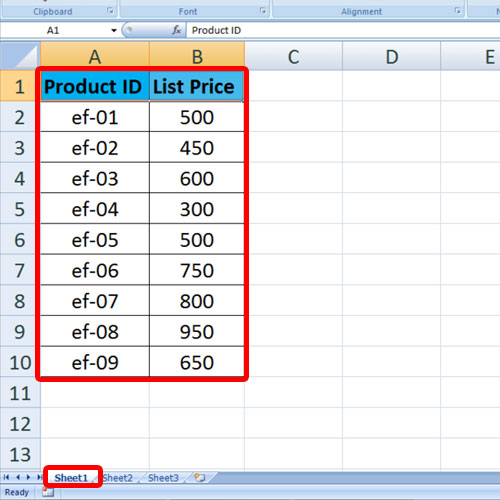Step 2 – Choose the current Sheet and Event handler

For that, we will open up the VBA editor window and select the sheet 1 module, since we want the function to be applied on the first sheet. Next, we will open up the object drop down menu and select the worksheet option. After that, we will set the event handler as “before double click”. This means that the function would be applied once we double click on the sheet.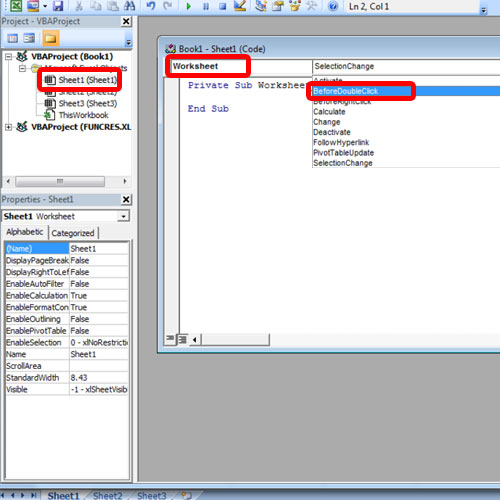Step 3 – Insert the Copy function

Next, we will start by defining the row from where the data is to be copied from.

After that, we will use the Do while loop to check if cells in row 2 of the first column contain any value. So as long as the product ID column is not empty, the rest of the tasks in the code will be carried out.

With that done, we will use the worksheets keyword and mention the sheet and the rows from where the data is to be copied.

Since we have defined the variable that represents the row to be copied in the very beginning, we will use that variable over here.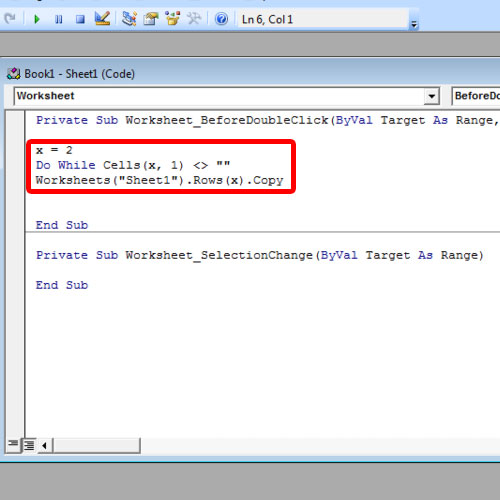Step 4 – Select the Range

Next, we will go to worksheet two and activate that. So for that, we will use the .Activate keyword.

After that, we will find out the first empty column in sheet 2 so that we can paste the data.

After that, we will paste the data in the active sheet, using the eRow keyword, which is basically the eRow that was found previously.

Once done we will type “Loop” over here, to end up the loop function.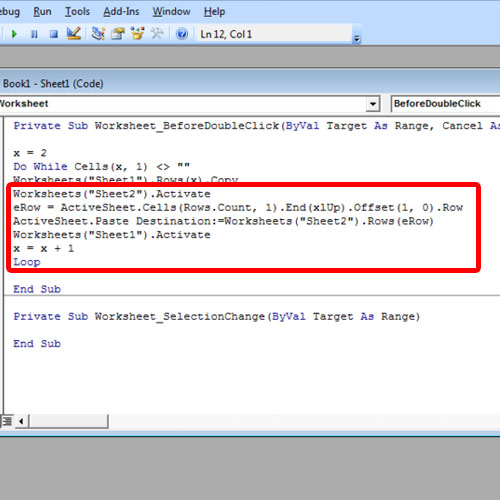Step 5 – Data pasted on another Sheet

Now let’s go back to the Excel Sheet and notice that when we double click on the sheet, the data will be copied and pasted in sheet 2.

And that’s basically how you can use VBA in excel to copy and paste data.